## Fluids

#### Divergence detected in AMG solver” in simulation of cavitating flow based on EoS equations

•Bart
Subscriber

I am conducting a simulation of cavitation in a simple geometry. However, i didn't use the cavitation models which had been implemented in Fluent. I am trying to implement a single-phase model (a barotropic models, pressure is only a function of density) into pressure based solver in Fluent by using UDFs. However, when the simulation was run, i got the error information about "Divergence detected in AMG solver".

The simple geometry is meshed with all-quadrilateral element.

I am using a Realizable k-epsilon turbulence model with scalable wall functions. Pressure-inlet of 30MPa and pressure-outlet of 1Mpa are adopt.

Validation of UDFs which is a piecewise function by an excel spreadsheet.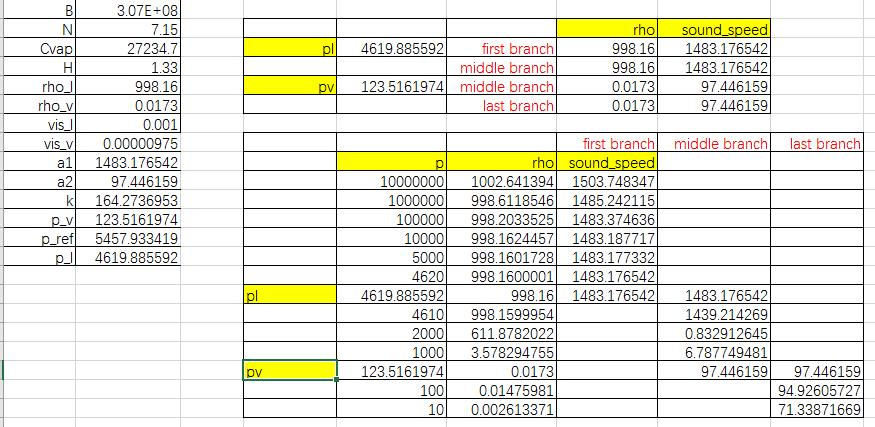The error is shown as follows:

When i checked the contour of density or pressure, there was a black cavity in the model.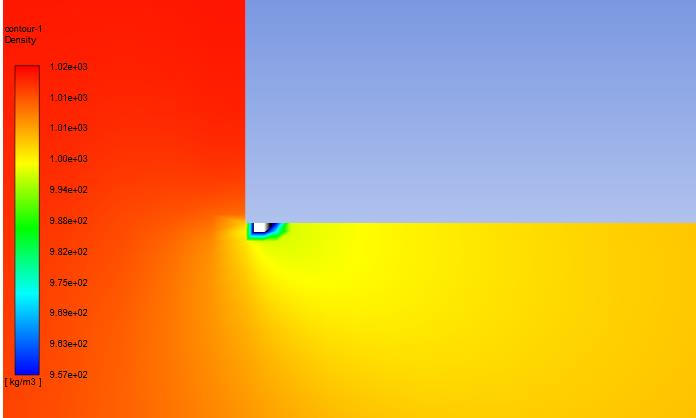•Rob
Ansys Employee

Turn the UDF off, does the model run? If so, focus on what the UDF is doing in the highlighted region.

•Bart
Subscriber

Thanks for your reply. If turn the UDFs off, the simulation is changed into a simple single-phase fluid. The model can run well, though the negative pressure appears due to the absence of cavitation model.

The content of UDF is a piecewise function (density = function (pressure)). And the funciton is continuous in terms of  the excel spreadsheet. Therefore i don't understand what you mean.

Thanks.

•Rob
Ansys Employee

So, what is the density in the negative pressure region if you plot on the graph?

•Bart
Subscriber

Actually, the occurance of negative pressure is wrong because the operation pressure is set to 0. Generally, in this case, the cavitation model can prevent pressure from falling below saturation vapor pressure.

In the case of UDF using, a pressure value corresponds to a density value. In this simulation, the error appears as before the pressure falls below the saturation vapor pressure. For example, as the min pressure is 100000 Pa, the calculation diverges and the corresponding density is changed into a black cavity as the graph shown. In this case, the negative pressure is forbidden. And sometimes AMG devergence occurs as the presence of negative pressure.

•Rob
Ansys Employee

Not exactly. Typically the solver sorts itself out within the time step as the pressure, density, volume etc are resolved. By adding in a UDF you may be hindering this process. Try running with a much lower dP and slowly increase this to test the UDF and models.

•Bart
Subscriber

I tried to fix the pressure-inlet as 50MPa and decrease gradually the pressure-outlet from 40MPa. As the pressure-out is 22MPa, the model can run well. While as the pressure-out is set to 21MPa, the devergence occurs.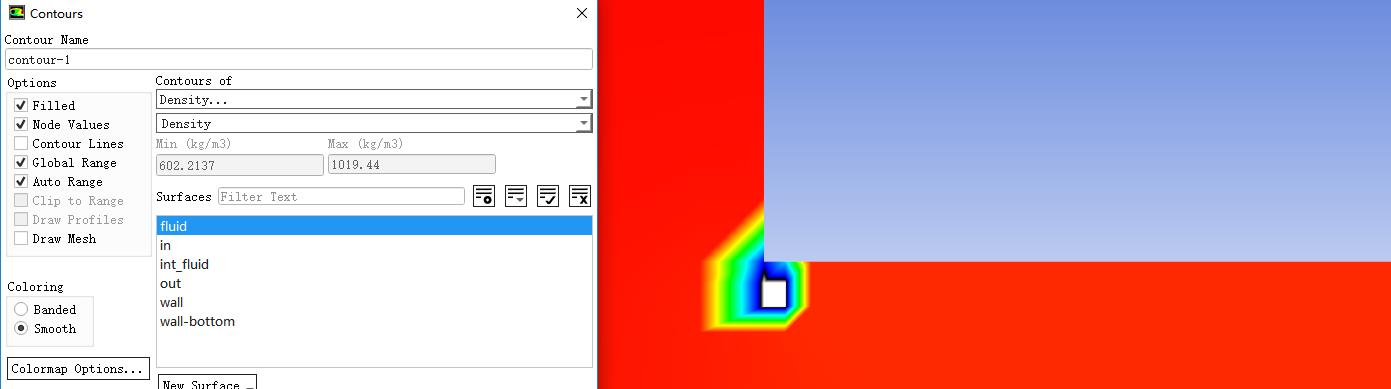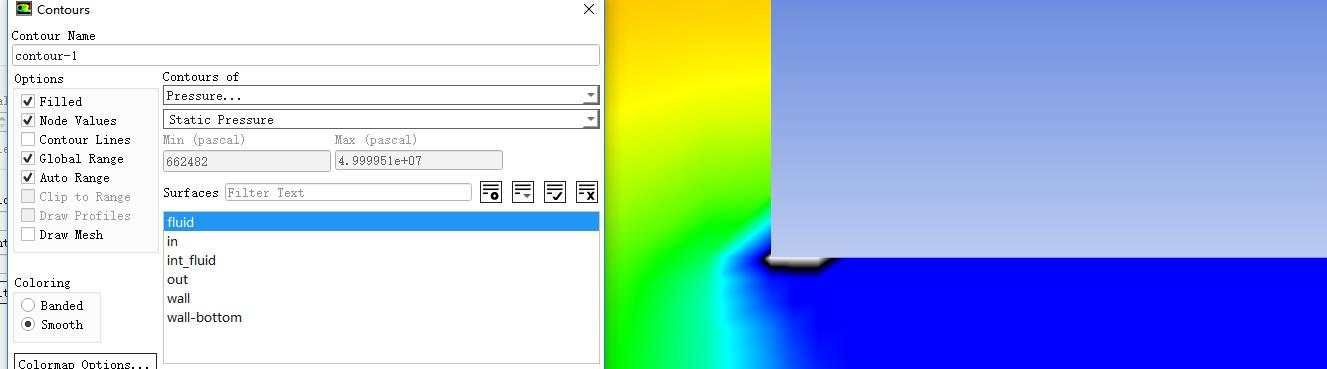Strangely, the Min density is 602 kg/m3, and the corresponding min pressure is 662482Pa. I can't understand this result because the density should be 998kg/m3 under the pressure of 662482Pa. A decrement of 1 MPa induces such big variation.

When i change the pressure-out from 25MPa to 20MPa, there is another error. The min pressure is 754074Pa and the density is 999kg/s. There is also a black cavity in the graph of density contour.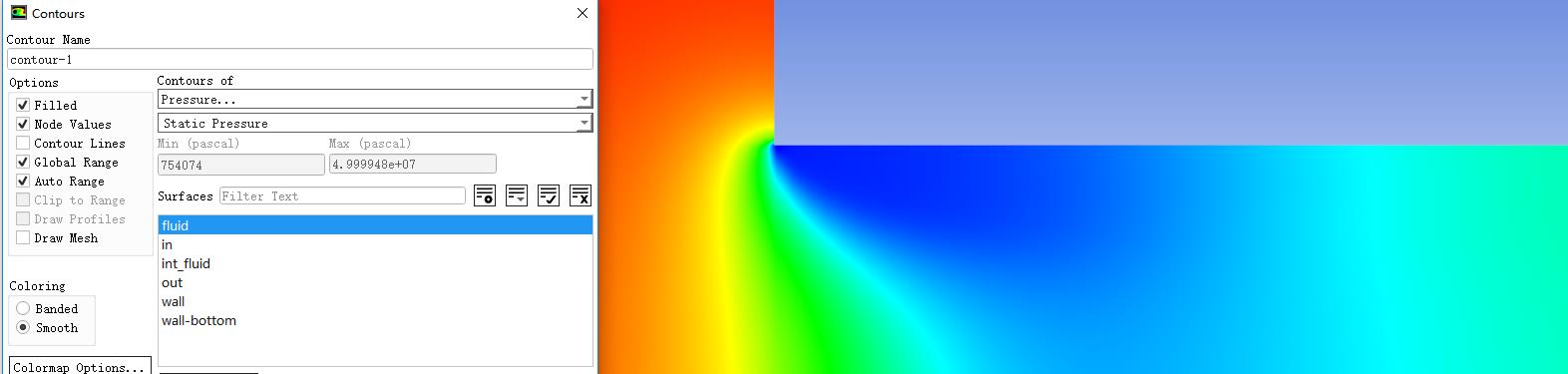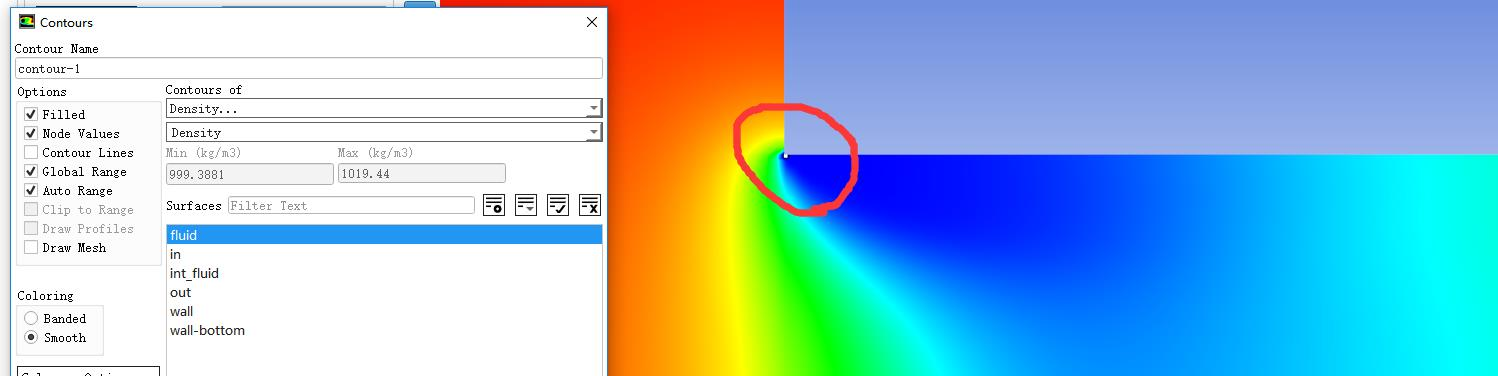I'm not sure if I need to make any changes in the multigrid panel.

Moreover, i read the HELP document. Updating properties (user-defined properties in my UDFs) is behind the equation solving. In this UDFs, the value of density is obtained from the value of pressure. Therefore, is there something wrong with the pressure solving or the AMG setup?

•Rob
Ansys Employee

Turn off node values & replot. You're looking at a smoothed result, but the solver will be using the cell values.

•Bart
Subscriber

I don't think it's a replot problem. The calculation diverges, then the graph obtained is wrong.

•Rob
Ansys Employee

Turn off node values & replot. You're looking at a smoothed result, but the solver will be using the cell values.   This may well give you a different range.

•Bart
Subscriber

I see. And now the result is shown

The calculation  diverges as the pressure is below 0(the operation pressure is 0). I have set a very small time step size.

•Bart
Subscriber

Hi, rwoolhou

I have tried many times. The simulation diverges as the first grid adjacent to the wall at the corner is below 0 Pa. Both a small URF and time step cannot solve this problem. I think the transition of pressure is too fast, but the grids at the corner are dense enough and y+ is ~0.5

Actually, i got a successful calculation of cavitation phenomenon at a small pressure difference in a bigger channel. However, the same setup failed under the condition of a larger pressure difference in a tiny channel.

I will be very appreciate if you can give further advices.

Kind regards.

•DrAmine
Ansys Employee
What about starting by smaller pressure differences than ramping it.
•Bart
Subscriber

I tried and it didn't work. Small pressure differences can make the calculation smooth. But, an increment of 0.1MPa can lead to the divergence as the injection pressure is 7MPa ans the outlet pressure is 3MPa. There is also a grid with negative pressure in the contour of pressure. Is there a special setup in multigrid panel that can solve my problem? Because i think the problem can be attributed to the processing mesh.

Kind regards.

•DrAmine
Ansys Employee
•Bart
Subscriber
•DrAmine
Ansys Employee
As you are using a barotropic model instead of the cavitation models please use udrgm for eos.
•DrAmine
Ansys Employee
Moreover switch on pseudo transient and start conservative probably you will need to be transient. Again for stability reasons I recommend using udrgm real gas.
•Bart
Subscriber

Firstly, what do the words "start conservative" mean?

Pseido transient will make the calculation diverge more fast. I tried to make the case transient, but it didn't work.

Moreover, UDRGM makes the energy model on and needs more equations, such as enthalpy, entropy, thermal_conductivity, which aren't provided in the published paper. And there is no example of UDRGM in users' guide that involved the phase transition.

I hope to use the model without energy equation. I think the large pressure difference at the corner makes the pressure term diverge. Is there any option to correct pressure term? Because I have a successful case in the model of large channel under small pressure difference based on the similar Reynolds number, I don't want to give up this.

Kind regards.

•DrAmine
Ansys Employee
Just switch off the energy equation keeping only the density dependent on pressure. I cannot comment on your density UDF as I do not know the paper (what is the ref). Try with unsteady solver and start with small time steps. Now I wish happy weekend
•Bart
Subscriber

Thank you very much! I will try to use UDRGM.

Finally, have a nice weekend!

Kind regards.